GFG App
Open AppBrowser
Continue

# SBI Clerk Prelims Reasoning Question Paper 2019

Directions (1-5): Study the information carefully and answer the questions given below.

Eight persons are sitting around a circular table, some are facing the centre while some facing outside the centre. U sits to the immediate left of X, who sits fourth to the left of W. Only one person sits between Z and T when counted from the right of Z. U is not an immediate neighbour of V. T faces outsides. Z sits second to the left of V. S sits third to the left V. S and Z face opposite directions to each other. Immediate neighbours of Z face the same direction, but not as Z. Y and U face inside. X does not face outside.

Solution(1-5):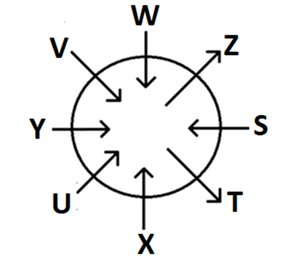Q1. How many persons are sitting between S and Y when counted from the right of Y?

a) Three

b) Two

c) Four

d) One

e) None

Solution.  a

Three persons are sitting between S and Y when counted from the right of Y.

Q2. Who among the following sits 3rd to the right of V?

a) Y

b) U

c) X

d) S

e) T

Solution. c

X sits 3rd to the right of V.

Q3. Four of the following five are alike in a certain way and hence form a group, find the one that does not belong to that group?

a) T

b) S

c) U

d) Y

e) V

Solution: a

T does not belong to that group.

Q4. Who sits second to the right of T?

a) Z

b) X

c) S

d) W

e) U

Solution.  e

U sits second to the right of T.

Q5. If X and W interchange their positions, then who sits second to the left of W?

A. U

B. Z

C. Y

D. S

E. None of these

Solution: c

If X and W interchange their positions, then Y sits second to the left of W.

Directions (6-8): Study the following information carefully and answer the questions given below:

Mr Raju started from point N, he walked 5km in the east direction to reach point S.  From point S, he turns 90 degrees in an anticlockwise direction and walked 4 km to reach point R. From point R, he takes a left turn and walks 5km to reach point M from where he turned 135 degrees towards his right and walked a certain distance to reach point P which is in line with point R and S.

Solution(6-8):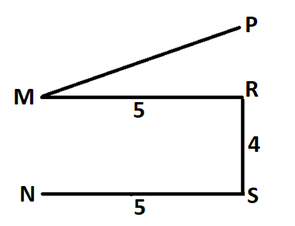Q6. What is the shortest distance between points P and S?

a) 9km

b) 8km

c) 13km

d) 15km

e) Cannot be determined

Solution: a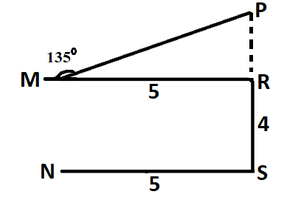The distance between R and M is the same distance between R and P as there is a 45-degree angle between R and P. The shortest distance between point P and S is 9km.

Q7.In which direction is the point P with respect to Point N?

a) North

b) South

c) East

d) Northeast

e) None of the above

Solution: d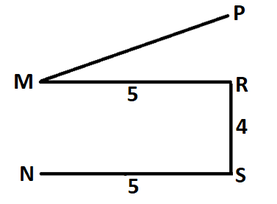Point P is in the northeast direction with respect to Point N.

Q8. In which direction is Point S with respect to Point M?

a) North

b) South

c) East

d) Northeast

e) None of the above

Solution: e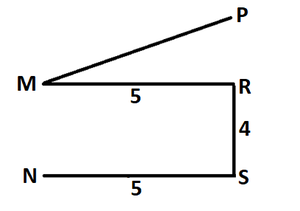Point S is in the southeast direction with respect to Point M.

Directions (9-13): Study the following information carefully and answer the question given below-

Eight friends i.e. D, G, H, K, L, M, N and P were born in different months of two days in the same year. They have born either on the 11th or 28th. The months are February, July, August and September.

There are three friends who have been born between L and M. P has born after L but on even days and he does not bear after M. G has born on even days but just before L. There is one friend who has born between P and K. K has not born just after L. M has born after August.  Not more than one friend has been born between G and D in which D has born before G.  H has born on an odd day. N has not born after August.

Solution(9-13):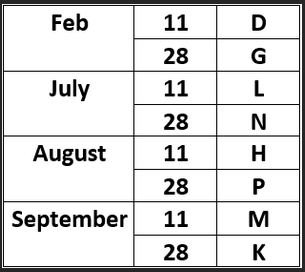Q9. Who among the following has born on 28th August?

a) L

b) K

c) P

d) M

e) None of these

Solution: c

P has born on 28th August.

Q10. How many friends have been born between D and H?

a) One

b) Two

c) Three

d) Five

e) Four

Solution: c

Three friends have born between D and H.

Q11. K has born on which day and month?

a) 28th September

b) 11th August

c) 28th August

d) 18th July

e) None of these

Solution: a

K has born on 28th September.

Q12. How many students have been born after N?

a) One

b) Two

c) Three

d) Five

e) None of these

Solution: e

Four students have born after N.

Q13. Who among the following has born on 11th February?

a) K

b) M

c) D

d) L

e) None of these

Solution: c

D has born on 11th February.

Q14. If in the word “PREDICT” all vowels are changed by the next letter and all consonants are changed by the previous letter of the English alphabetical series. Then. How many letters are repeated?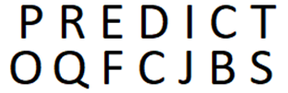a) None

b) One

c) Two

d) Three

e) None of these

Solution: a

No letters are repeated.

Direction (15-19): Study the following information carefully and answer the questions given below:

Eight students i.e. A, B, C, D, W, X, Y and Z live in ‘Shiva Hostel’ which has a total of eight floors. The ground floor is numbered 1, while the topmost floor is numbered 8.

There are three floors between the floor on which X and Clive. C lives below X who lives on an odd-numbered floor. W lives on an odd-numbered floor but above the floor on which X lives. Z does not live on an odd-numbered floor. Life just above the floor on which Z lives. B lives above the floor on which D lives but not on the topmost floor.

Solution(15-19):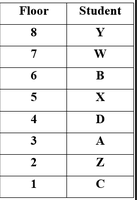Q15. On which floor, D live?

a) 3rd

b) 2nd

c) 4th

d) 7th

e) 1st

Solution: c

D lives on the 4th floor

Q16. Who among the following lives on the ground floor?

a) W

b) B

c) Z

d) C

e) D

Solution: d

C lives on the ground floor.

Q17. How many students live between the floor on which A and D live?

a) None

b) One

c) Two

d) Four

e) None of these

Solution: a

No one lives between A and D.

Q18. How many students live above the floor on which B lives?

a) None

b) One

c) Two

d) Four

e) None of these

Solution: c

Two students live above the floor on which B lives.

Q19. If Z interchanges his floor to B’s floor, then who among the following lives just above the floor on which Z lives?

a) W

b) Y

c) A

d) C

e) X

Solution: a

W lives just above the floor on which Z live.

Direction (20-22): In each of the following questions assuming the given statements to be true, find which of the

two conclusions I and II given below them is/are definitely true and give your answer accordingly.

(a) If only conclusion I is true

(b) If only conclusion II is true

(c) If either conclusion I or II is true

(d) If neither conclusion I nor II is true

(e) If both conclusions I and II are true

Q20. Statements:

Z<U≥W, Y≥P>R=Z,

Conclusions:

I. W>P

II.Y>Z

Solution: b

Conclusions:

I. W>P(False)

II.Y>Z(True)

If only conclusion II is true

Q21. Statements:

A>P<C≥M>E, E>G=N>L

Conclusions:

I. M>N

II.P≥L

Solution: a

Conclusions:

I. M>N(True)

II.P≥L(False)

If only conclusion I is true

Q22. Statement:

Z > I < Y> K, P > I < V < E = J

Conclusion:

I. E < Z

II. P < Y

Solution: d

Conclusion:

I. E < Z(False)

II. P < Y(False)

If neither conclusion I nor II is true

Direction (23-26): Study the following series carefully and answer the questions given below

792         652      748       936        521

Q23. If the second and third digits of each number are interchanged, how many numbers become the perfect cube?

a) None

b) 1

c) 2

d) 3

e) 4

Solution: c

2 numbers become the perfect Cube.

512 and 729 are the two numbers that form cubes of 8 and 9.

Q24. If in each number, the odd digits are subtracted by one and the even digits are added by One then, how many numbers are there in the new arrangement with a minimum of two repeated digits?

a) 2

b) 1

c) 4

d) 3

e) None

Solution: e In this case, All digits are different in number. So no digit will ever repeat. No digits are repeated in the new arrangement.

Q25. If all digits are arranged in reverse order within the number then, what will be the sum of the second and third digits of the number which is fourth number from the left in the new arrangement?

a) 9

b)6

c) 12

d) 11

e) None of these

Solution: c

After reverse order 639 is the fourth number from the left end. The sum of the second and third digits is 12.

Q26. If all the numbers are arranged in descending order from right to left then, what will be the Multiplying of all the three digits of the number which is fourth from the left in the new arrangement?

a) 126

b) 60

b) 224

d) 162

e) None of these

Solution: a

all the numbers are arranged in descending order from right to left then,

521      652      748      792        936

792 is fourth from the left end in the new arrangement. The Multiplying of all the three digits of the number is 126.

Directions (27-30): In each of the questions below. Some statements are given followed by conclusions/group of conclusions. You have to assume all the statements to be true even if they seem to be at variance from the commonly known facts and then decide which of the given conclusions logically follow from the information given in the statements:

a) If only conclusion I follows

b) If only conclusion II follows

c) If either I or II follows

d) If neither I nor II follows

e) If both I and II follows

Q27. Statements:

Only a few road are house.

Minimum house are town.

Conclusions:

I. All car are house.

II. No house is car.

a) If only conclusion I follows

b) If only conclusion II follows

c) If either I or II follows

d) If neither I nor II follows

e) If both I and II follows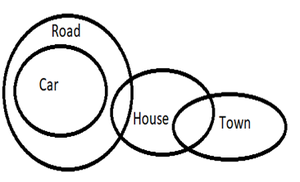Solution: d

If neither I nor II follows

Q28. Statements:

Some S are H.

All B are S.

No B is V.

Conclusions:

I. Some S can never be V.

II. Some V can be H.

a) If only conclusion I follows

b) If only conclusion II follows

c) If either I or II follows

d) If neither I nor II follows

e) If both I and II follows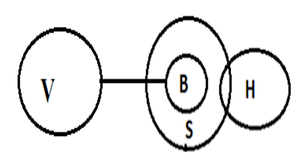Solution: e

If both I and II follows

Q29. Statements:

All Flower is Beautiful.

No Beautiful is Fruit.

Every Fruit are Seed.

Conclusions:

I. All beautiful being Seed is a possibility.

II. Some Seed being Flower is a possibility.

a) If only conclusion I follows

b) If only conclusion II follows

c) If either I or II follows

d) If neither I nor II follows

e) If both I and II follow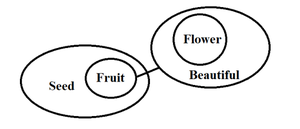Solution: e

If both I and II follow

Q30. Statements:

No Mat is Rat.

Only a few Tiger is King.

No Rat is Tiger.

Conclusions निष्कर्ष:

I. All King can never be Mat.

II. All Rat can never be King.

a) If only conclusion I follows

b) If only conclusion II follows

c) If either I or II follows

d) If neither I nor II follows

e) If both I and II follows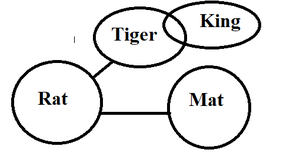Solution: d

If neither I nor II follows.

Directions (31-35): Study the following information carefully and answer the question given below-

Seven persons i.e. U, Y, Z, V, W, T and X have decided to go to different cities of the world on different days of the week starting from Tuesday to Monday. These cities are France, Singapore, China, Russia, Malaysia, Rome and New York. There are two days gap between W and X. Z goes to France but does not go after W.  Y goes after W but not to New York and China. U goes to Russia but before X. W goes to Singapore but after Friday. The one who goes to Rome goes just before Z. There are two days gap between Z and Y. T does not go before U. V goes after T but not to China. X does not go to New York and China. V does not go on Saturday and Sunday.

Solution(31-35):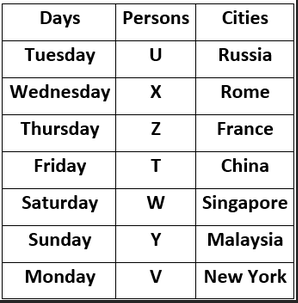Q31.  Who among the following goes to Malaysia?

a) V

b) U

c) T

d) W

e) Y

Solution: e

Y goes to Malaysia.

Q32. How many people go to different cities before Z?

a) One

b) Two

c) Three

d) Four

e) None of these

Solution: b

Two persons go to different cities before Z.

Q33. T goes to which city?

a) China

b) New York

c) France

d) Rome

e) Russia

Solution: a

T goes to China.

Q34. Who among the following goes on Friday?

a) V

b) F

c) T

d) Z

e) X

Solution: c

T goes on Friday.

Q35. Who among the following goes on Tuesday?

a) X

b) U

c) Y

d) V

e) W

Solution: b

U goes on Tuesday.

My Personal Notes arrow_drop_up